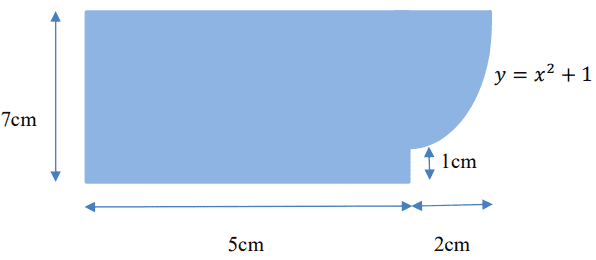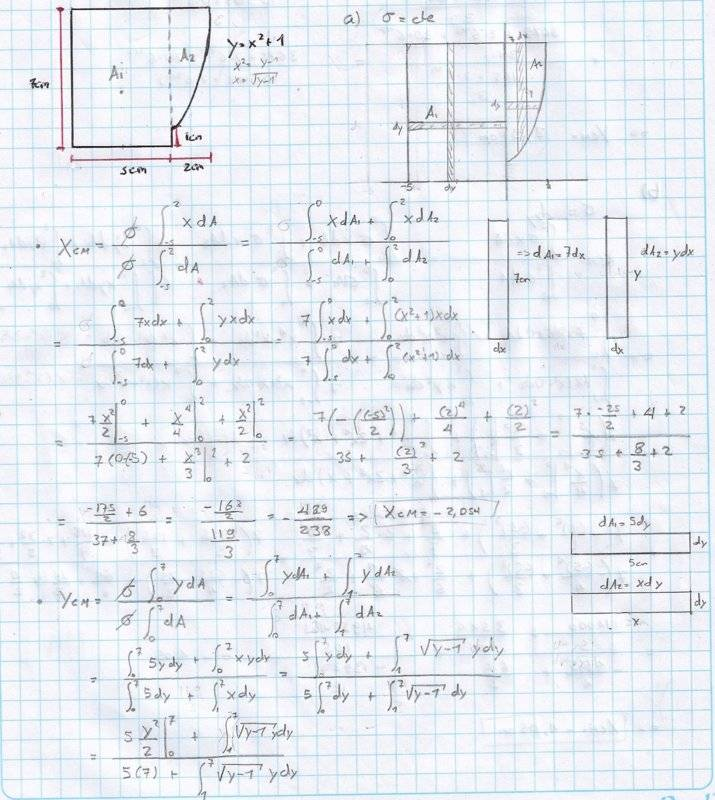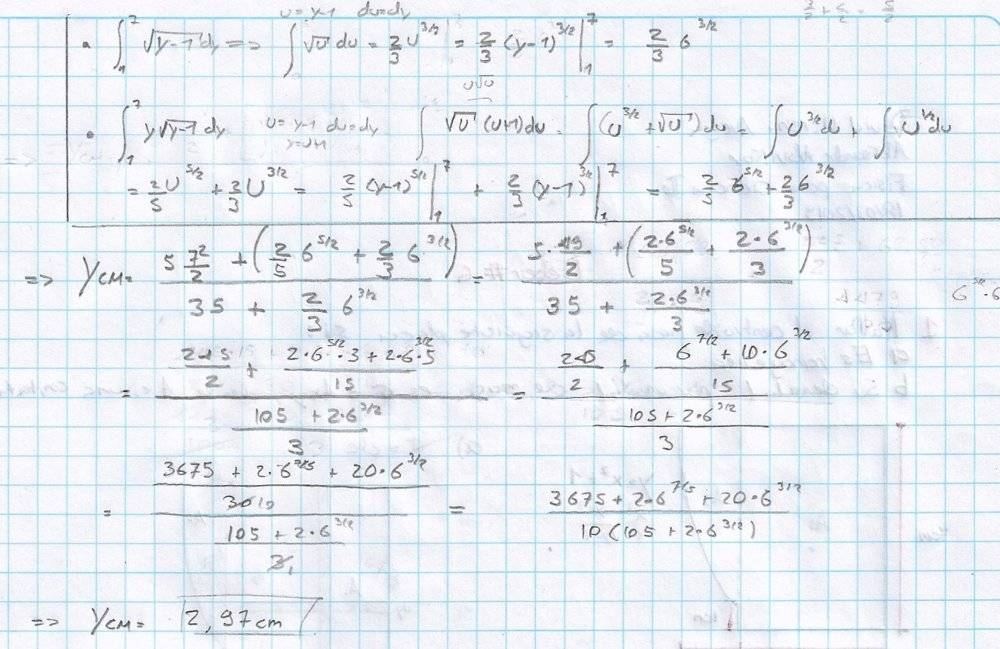# Find the center of mass of a plate

Tags:
1. Mar 16, 2019 at 7:21 PM

### Davidllerenav1. The problem statement, all variables and given/known data
Find the center of mass of the next plate if:
A) Is homogeneous
B) Its density per unit mass is $\sigma=Axy$, where $A$ is a constant.2. Relevant equations
$X_{cm}=\frac{\int\sigma x dA}{\int\sigma dA}$
$Y_{cm}=\frac{\int\sigma y dA}{\int\sigma dA}$
3. The attempt at a solution
I did part A) as is shown in the picture below:Am I correct?